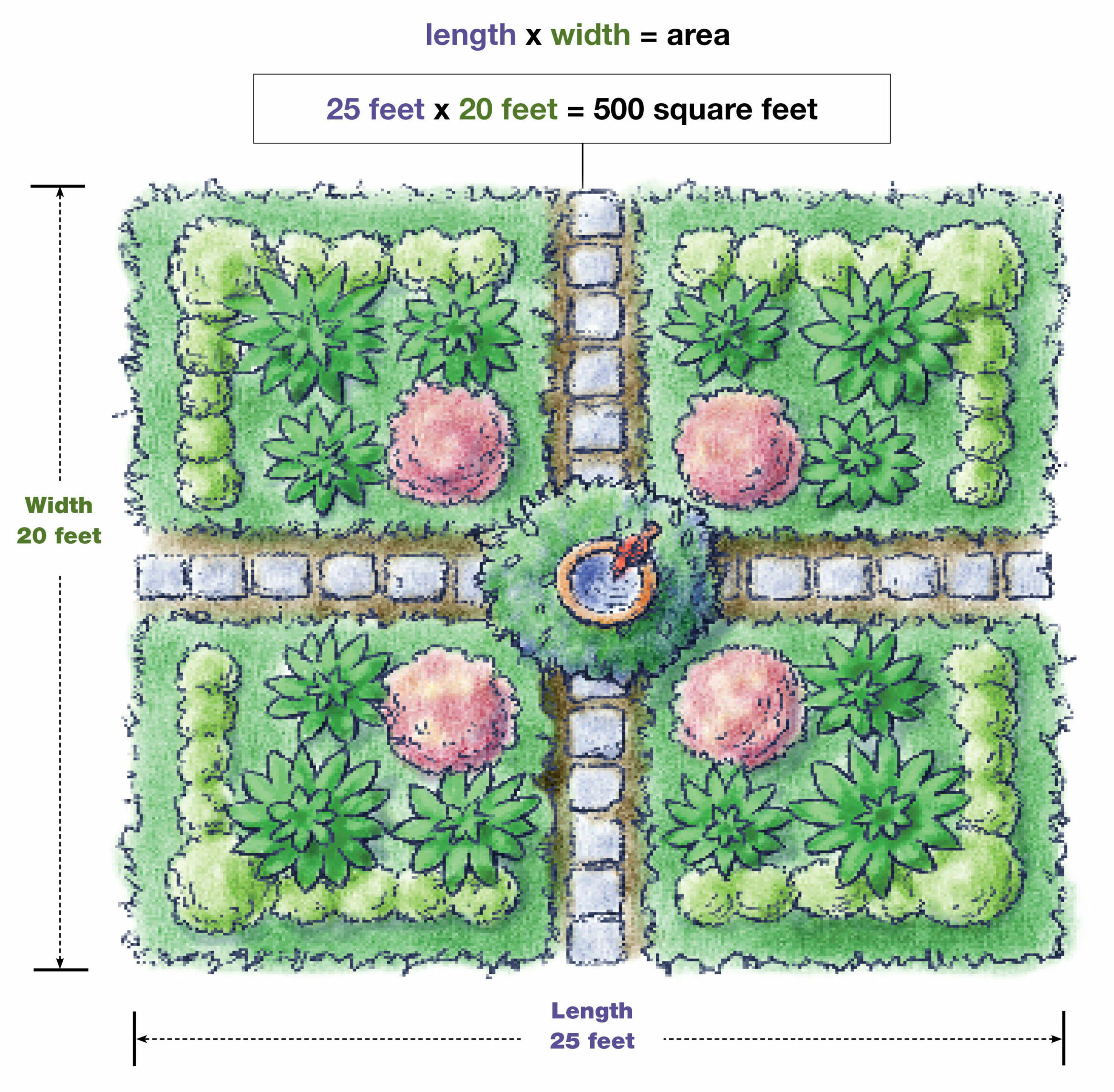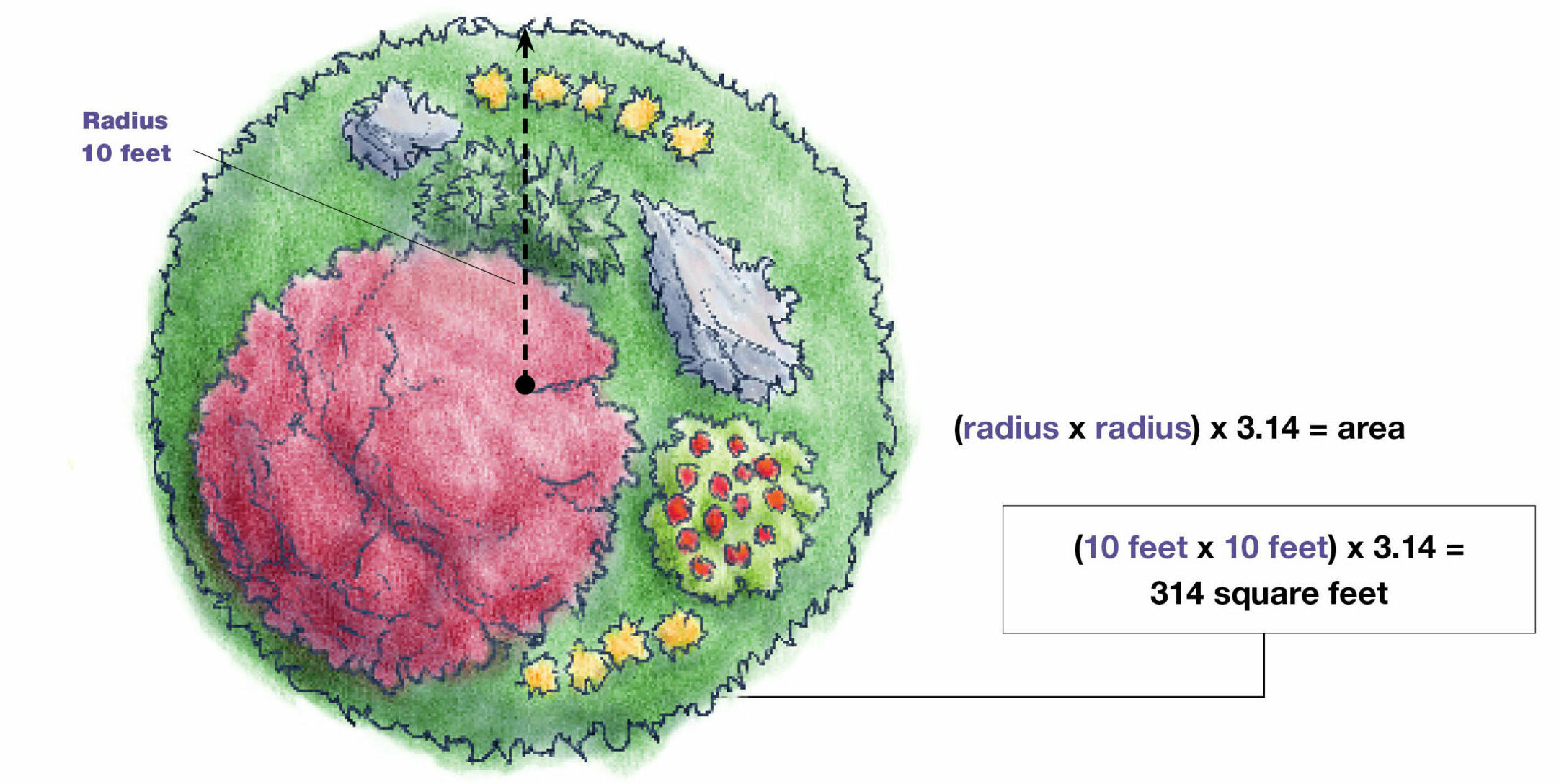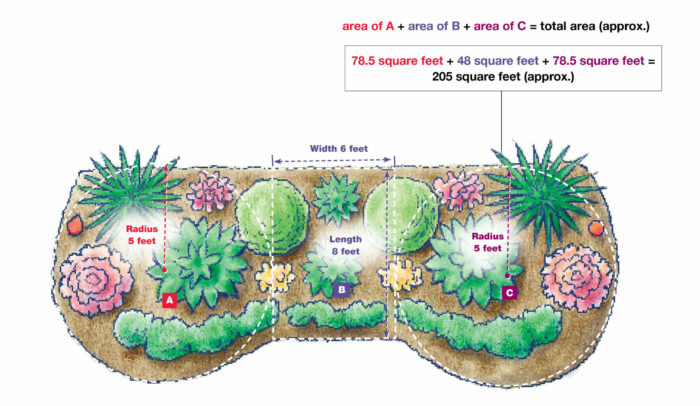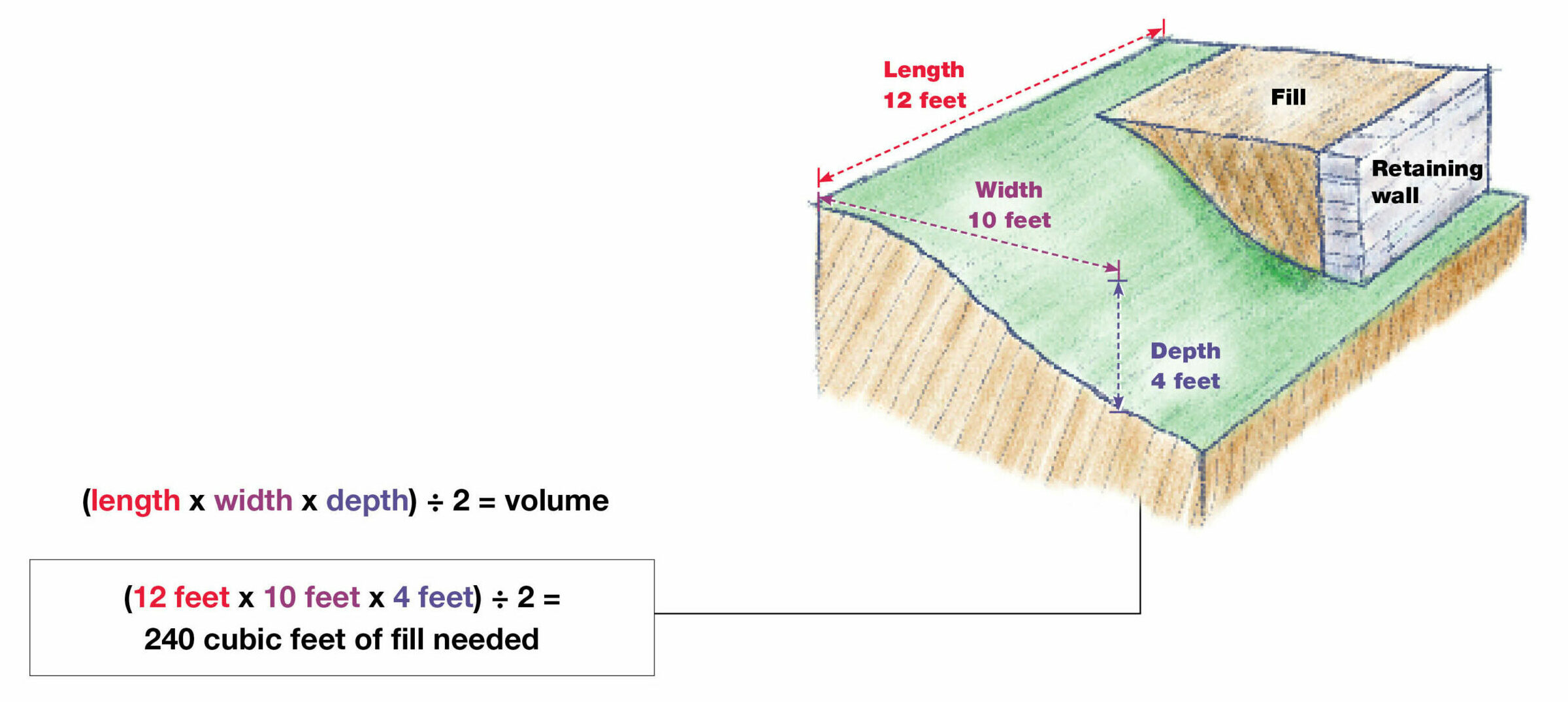How-To

# Garden Math: Calculating the Area and Depth of Your Garden

## Save time and money with simple equations to calculate what your garden needs

From fertilizer to mulch and paving stones to plants, too much of a good thing can mean, at best, unused material stored in the garage and, at worst, dead plants.

It can also mean that you have spent money you did not need to spend. On the flip side, finding that your paths look unkempt because you under­estimated how much mulch you needed can be discouraging, as can languishing plants—despite what you thought were carefully measured fertilizer applications. Whether you are a math wiz or have a math phobia, learning some simple calculations to use for common gardening situations can help you save time and money while you turn your garden into an Eden.

Learn more: 8 Ways to Save Money at the Garden Center

Here are some basic calculations that will help you figure out what you need.

## Calculating the square footage of your garden

 What is it? Square footage is the measurement of a flat, two-dimensional space (for example, a patio, a perennial bed, or a deck). When do I need it? You need to know square footage when purchasing materials to cover or build a flower bed, a patio, or a deck, for instance when purchasing flagstone, pavers, sod, or fertilizer.

### ♦ Finding the square footage of a square or rectangular area of land

Multiply the length by the width to determine the square footage—or area—of a square or rectangle.Find the square footage by multiplying the length and width of the area in question. Make sure to keep your units the same (feet or inches).

### ♦ Finding the square footage of a triangular area of land

• Consider the longest side of the triangle the base.
• Measure a perpendicular line from the opposite angle to the base to determine the triangle’s height.
• Multiply the base by the height, and divide that number in half to determine the area.### ♦ Finding the square footage of a circular piece of land

• Find the center point of the circle.
• Measure from that point to the edge of the circle to determine the radius.
• Multiply the radius by itself.
• Multiply that number by pi (3.14) to find the area of your circle.Square the radius—that is, multiply the radius of the circle times itself—and then multiply the resulting number by pi, which is 3.14.

### ♦ Square footage of an irregular shape

• Break up the space into regular shapes (squares, rectangles, triangles, and circles).
• Determine the area of each shape.
• Add the areas of each shape to come up with an approximate area of the bed.Break irregular shaped areas into its component parts, calculate the area for each individual section, and then add them together.

## Finding the cubic footage of your garden

 What is it? Cubic footage is a measurement of three-dimensional volume, which is important to know when you need material that will be applied to a certain depth or thickness. When do I need it? You will need to know the cubic footage of your area if you are ordering materials such as topsoil, mulch, or compost.

### ♦ To cover a level area

• If the thickness or depth of your material is measured in inches, convert it to feet.
• Determine the square footage of the space.
• Multiply the square footage by the desired depth of the material to determine the cubic footage—or volume.Multiply the length, width, and depth of the area you need to cover.

### ♦ To level a sloped area

• Determine the length of the area you would like to level.
• Create a level line that extends out from the top of the slope and stops at a point directly above the bottom of the slope. This line represents the width of the area.
• Create a perpendicular line from the end of the width line to the bottom of the slope. This line represents the depth of the area.
• Multiply the length by the width by the depth to determine the cubic footage of the entire area.
• Divide that number in half (to account for the soil that is already there) to determine how much fill you will need.Since you don’t need to fill in the entire area, you divide by 2, and get the approximate amount of fill needed to level off the slope. You may need to adjust this depending on how much of your slope is level.

Tip: Sometimes material sold in large quantities is measured in cubic yards. To convert cubic feet into cubic yards, divide the number of cubic feet by 27 (because there are 27 cubic feet in 1 cubic yard).

Patrick Beam plays with math on a daily basis for his professional landscaping business in Columbus, Ohio.

#### Gardening Basics

##### Gardening Basics

Expert advice for the beginner

Become a member and get unlimited site access, including the Gardening Basics Project Guide.

# Fine Gardening Magazine